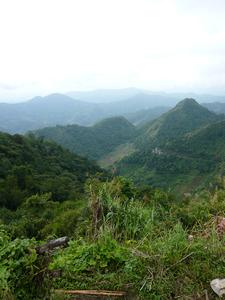# Calculate the area of an image using Matplotlib

• Last Updated : 31 Jul, 2021

Let us see how to calculate the area of an image in Python using Matplotlib.

Algorithm:

1. Import the matplotlib.pyplot module.
2. Import an image using the imread() method.
3. Use the shape attribute of the image to get the height and width of the image. It fetches the number of channels in the image.
4. Calculate the area as, area = height * width.
5. Display the area.

Example 1: Consider the following image :“GFG.jpg”

## Python3

 `# import necessary library``import` `matplotlib.pyplot as plt` `# read an image``img ``=` `plt.imread(``"GFG.jpg"``)` `# fetch the height and width``height, width, _ ``=` `img.shape` `# area is calculated as “height x width”``area ``=` `height ``*` `width` `# display the area``print``(``"Area of the image is : "``, area)`

Output :

`Area of the image is : 50244`

Example 2: Consider the following image :“image.jpg”

## Python3

 `# import necessary library``import` `matplotlib.pyplot as plt` `# read an image``img ``=` `plt.imread(``"image.jpg"``)` `# fetch the height and width``height, width, _ ``=` `img.shape` `# area is calculated as “height x width”``area ``=` `height ``*` `width` `# display the area``print``(``"Area of the image is : "``, area)`

Output :

`Area of the image is : 213200`

My Personal Notes arrow_drop_up